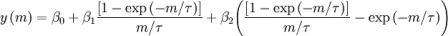Want to share your content on R-bloggers? click here if you have a blog, or here if you don't.

In a recent post (here, by @teramonagi), Teramonagi mentioned the use of PCA to model yield curve, i.e. to obtain the three factor,parallel shift“, “twist” and “butterfly“. As in Nelson & Siegel, if m is maturity,$yleft( m right)$ is the yield of the curve at maturity m, assume thatwhere β0, β1, β2 and τ, are parameters to be fitted

• β0 is interpreted as the long run levels of interest rates (the loading is 1, it is a constant that does not decay)
• β1 is the short-term component (it starts at 1, and decays monotonically and quickly to 0);
• β2 is the medium-term component (it starts at 0, increases, then decays to zero);
• τ is the decay factor: small values
produce slow decay and can better fit the curve at long maturities,
while large values produce fast decay and can better fit the curve at
short maturities; τ also governs where β2 achieves its maximum.

(see e.g. here). Those factors can be obtained using PCA,

```term.structure = read.csv("C:\tmp\FRB_H15.csv",
stringsAsFactors=FALSE)
term.structure = tail(term.structure,1000)
term.structure = term.structure[,-1]
label.term = c("1M","3M","6M","1Y","2Y","3Y","5Y"
,"7Y","10Y","20Y","30Y")
colnames(term.structure) = label.term
term.structure = subset(term.structure,
term.structure\$'1M' != "ND")
term.structure = apply(term.structure,2,as.numeric)
term.structure.diff = diff(term.structure)
term.structure.princomp = princomp(term.structure.diff)
"Second principal component","Third principal component")
par(xaxt="n")
par(xaxt="s")
axis(1,1:length(label.term),label.term)
> summary(term.structure.princomp)
Importance of components:
Comp.1    Comp.2     Comp.3     Comp.4     Comp.5      Comp.6      Comp.7      Comp.8
Standard deviation     0.2028719 0.1381839 0.06938957 0.05234510 0.03430404 0.022611518 0.016081738 0.013068448
Proportion of Variance 0.5862010 0.2719681 0.06857903 0.03902608 0.01676075 0.007282195 0.003683570 0.002432489
Cumulative Proportion  0.5862010 0.8581690 0.92674803 0.96577411 0.98253486 0.989817052 0.993500621 0.995933111```

using Teramonagi’s
R code,When
generating stress scenarios, the idea is to generate independently
those factors (or components) and then to aggregate them (using the expression given above). With Principal Component Analysis, PCA, we get orthogonal components, while with Independent Component Analysis, ICA, we get independent components. And independence and null correlation can be rather different. We recently discussed that idea in a paper with Christophe Villa (available soon here).

Consider the following sample

```ns=10000
X=runif(ns)
Y=runif(ns)
I=(Y<.25)*(Y<3*X)*(Y>X/3) +
(Y>.75)*(Y3*X-2)+
(Y>.25)*(Y<.75)*(Y<3*X)*(Y>3*X-2)
FACT1=X[I==1]
FACT2=Y[I==1]
DATA=data.frame(FACT1,FACT2)
PCA<-princomp(DATA)
op <- par(mfrow = c(1, 2))
plot(FACT1[1:2000],FACT2[1:2000],main="Principal component analysis",col="black",cex=.2,xlab="",ylab="",xaxt="n",yaxt="n")
arrows(.5,.5,.8,.8,type=2,col="red",lwd=2)
arrows(.5,.5,.2,.8,type=2,col="red",lwd=2)
plot(PCA\$scores,cex=.2,main="Principal component analysis",
xaxt="n",yaxt="n")```

The PCA obtain the following projections on the two components (drawn in red, below)

```> X=PCA\$scores[,1];
> Y=PCA\$scores[,2];
> n=length(FACT1)
> x=X[sample(1:n,size=n,replace=TRUE)]
> y=Y[sample(1:n,size=n,replace=TRUE)]

Comp.1 Comp.2
FACT1 -0.707  0.707
FACT2 -0.707 -0.707

Comp.1 Comp.2
Proportion Var    0.5    0.5
Cumulative Var    0.5    1.0

> F1=0.707*x-.707*y
> F2=0.707*x+.707*y```Hence, with PCA, we have two components, orthogonal, with a triangular distribution, so if we generate them independently, we obtainwhich is quite different, compared with the original sample. On the other hand, with ICA,we obtain factors that are really independent....

```library(fastICA)
nt=10000
ICA<-fastICA(DATA,2)
m=ICA\$K
x=ICA\$S[,1]
y=ICA\$S[,2]
plot(ICA\$S,cex=.2,main="Independent component analysis",
xlab="",ylab="",xaxt="n",yaxt="n")```

see below for the graphs, and here for more details,